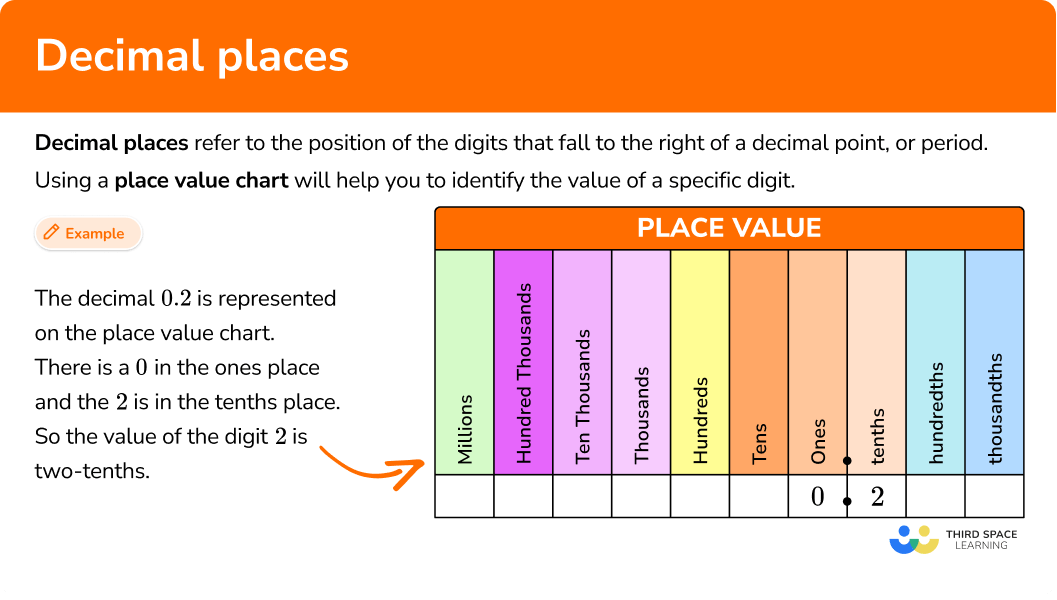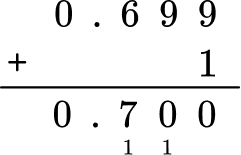# Decimal places

Here you will learn about decimal places, including how to round numbers to the tenths place, hundredths place and thousandths place.

Students will first learn about decimal places as part of number and operations in base ten, in elementary school.

## What are decimal places?

Decimal places refer to the position of the digits that fall to the right of a decimal point, or period.

The first decimal place to the right of the decimal point is the tenths place.

The second decimal place is the hundredths place.

The third decimal place is the thousandths place.

The decimal system continues up to the ten-thousandths place, the hundred-thousandths place, the millionths place and beyond.

• Using a place value chart will help you to identify the value of a specific digit.

For example,

The decimal 0.2 is represented on the place value chart.

Notice that there is a 0 in the ones place and the 2 is in the tenths place.

So the value of the digit 2 is two-tenths.

Using additional zeros in the place value chart won’t change the value of the decimal, but would change the decimal place and how you read the decimal.

0.20 can be read as twenty hundredths.

0.200 can be read as two-hundred thousandths

These values are all equivalent.

• You can use place value to round a number to given decimal places such as tenths, hundredths, and thousandths.

To round a decimal, you find the decimal place you wish to round to and look at the value after that number. If the value after the chosen place value is a 5 or above you round up, if it is less than 5, you round down.

It is important to give the number of digits required after the decimal point, even if the last digit would be a zero.

For example,

Round 19.237 to the nearest tenth.

19.{\color{orange}2¦}{\color{blue}3}7

3 is less than 5, so we’d leave the 2 as it is.

19.237 rounded to the nearest tenth would be 19.20.

### What are decimal places?## Common Core State Standards

How does this relate to 4th grade math and 5th grade math?

• Grade 5: Number and Operations in Base Ten (5.NBT.A3)
Read, write, and compare decimals to the thousandths

• Grade 5: Number and Operations in Base Ten (5.NBT.A4)
Use place value understanding to round decimals to any place

## How to round to decimal places

In order to round to a decimal place with the rule:

1. Find the rounding place and look at the digit immediately to the right of the rounding place.
2. When that digit is \bf{5} or greater, add \bf{1} to the rounding digit. When it is less than \bf{5} , leave the rounding digit alone.
3. Drop all the digits to the right of the rounded digit.
4. Write the rounded number.

## Decimal place examples

### Example 1: rounding to the tenths place

Round 36.248 to the nearest tenth.

1. Find the rounding place and look at the digit immediately to the right of the rounding place.

You need to locate the tenths place, the first digit to the right of the decimal point.

2When that digit is \bf{5} or greater, add \bf{1} to the rounding digit. When it is less than \bf{5} , leave the rounding digit alone.

4 is less than 5.

As 4 is less than 5, you round down.

3Drop all the digits to the right of the rounded digit.

4Write the rounded number.

36.248 is \bf{36.2} to the nearest tenth.

### Example 2: rounding to the tenths place

Round 7.97 to the nearest tenth.

Find the rounding place and look at the digit immediately to the right of the rounding place.

When that digit is \bf{5} or greater, add \bf{1} to the rounding digit. When it is less than \bf{5} , leave the rounding digit alone.

Drop all the digits to the right of the rounded digit.

Write the rounded number.

### Example 3: rounding to the hundredths place

Round 97.071 to the nearest hundredth.

Find the rounding place and look at the digit immediately to the right of the rounding place.

When that digit is \bf{5} or greater, add \bf{1} to the rounding digit. When it is less than \bf{5} , leave the rounding digit alone.

Drop all the digits to the right of the rounded digit.

Write the rounded number.

### Example 4: rounding to the hundredths place

A calculator displayed an answer to a calculation as 53.298. What is this value rounded to the nearest hundredths place?

Find the rounding place and look at the digit immediately to the right of the rounding place.

When that digit is \bf{5} or greater, add \bf{1} to the rounding digit. When it is less than \bf{5} , leave the rounding digit alone.

Drop all the digits to the right of the rounded digit.

Write the rounded number.

### Example 5: rounding to the thousandths place

Round 14.9792 to the nearest thousandth.

Find the rounding place and look at the digit immediately to the right of the rounding place.

When that digit is \bf{5} or greater, add \bf{1} to the rounding digit. When it is less than \bf{5} , leave the rounding digit alone.

Drop all the digits to the right of the rounded digit.

Write the rounded number.

### Example 6: rounding to the thousandths place

Round 0.0715 to the nearest thousandth.

Find the rounding place and look at the digit immediately to the right of the rounding place.

When that digit is \bf{5} or greater, add \bf{1} to the rounding digit. When it is less than \bf{5} , leave the rounding digit alone.

Drop all the digits to the right of the rounded digit.

Write the rounded number.

### Teaching tips for decimal places

• Allowing students to use a place value chart when first studying decimal places, allows them to visualize how each number relates with the place value names and positions.

• Hands-on decimal activities, including using manipulatives, allows students to explore the decimal system in an impactful way.

• Number lines are a great place to start students when first learning how to round. The rule makes more sense once they’ve had the opportunity to see how rounding happens. Not giving students the opportunity to use the number line can lead to misconceptions and mistakes later down the line.

### Easy mistakes to make

• Adding \bf{1} to all of the previous digits when rounding up
A common error when rounding up is to increase all of the digits to the left by 1.
For example, if 53.683 was rounded to the tenths place, a student may write 64.7 instead of the correct answer 53.7.

• Decreasing the value of the rounding digit when rounding down
When rounding down, the value of the rounding digit remains the same, it does not decrease. For example, round 5.24 to the nearest tenth. The 4 tells us to round down, so the rounding number would stay the same, 5.2, not decrease to 5.1.

• Leaving out zeros when rounding a \bf{9} up
It is important to remember to write the number of decimals to the place value given. For example, if 23.997 is rounded to the hundredths place, it would be written as 24.00.

• Mixing up whole numbers and decimals
It is confusing to some when trying to determine the difference between decimal place value: thousandths, hundredths and tenths with whole numbers place value: thousands, hundreds and tens. Consistent practice with these terms will help with familiarity.

### Decimal places practice questions

1. Round 5.162 to the nearest tenth.

5.15.26.25.0The digit in the tenths place is 1. The value to the right of this is 6, which is greater than 5, so round up.

2. Round 36.715 to the nearest tenth.

4036.836.737.7The digit in the tenths place is 7. The value to the right of this is 1, which is less than 5, so round down.

3. Round 21.734 to the nearest hundredth.

21.7421.7321.8321.80The digit in the hundredths place is 3. The value to the right of this is 4, which is less than 5, so round down.

4. Round 1.7368 to the nearest hundredth.

1.700.741.741.84The digit in the hundredths place is 3. The value to the right of this is 6, which is greater than 5, so round up.

5. Round 0.6998 to the thousandths place.

0.7000.6990.70.600The digit in the thousandths place is 9 and the value to the right of this is 8. \, 8 is greater than 5 so round up – increasing 9 by 1 gives us 10, so you need to increase the previous 9 by 1 which also gives us 10.

Therefore you need to increase the 6 by 1 to give us 7.You need to include the zeros in our answer so that it is written to the thousandths place.

6. Round 0.03197 to the nearest thousandth.

0.0310.0400.1420.032The digit in the thousandths place is 1. The value to the right of this is 9, which is greater than 5, so round up.

## Decimal places FAQs

What decimals should I expect my students to know and work with?

Following the Common Core recommendations, in 5th grade, students should work with tenths place, hundredths place and thousandths place. Check your state’s specific standards for further clarification.

Why is learning about decimal places important?

Decimals are used in everyday life, including money and measurements. Decimals provide a more precise number that are needed in some situations.

What is “decimal place value”?

Decimal place values are all the digits in a decimal number. A tenth is the first digit to the right of a decimal point. The second digit to the right of a decimal point is the hundredths place and the third is the thousandths place. Decimals can also include ten thousandths and even millionths.

## Still stuck?

At Third Space Learning, we specialize in helping teachers and school leaders to provide personalized math support for more of their students through high-quality, online one-on-one math tutoring delivered by subject experts.

Each week, our tutors support thousands of students who are at risk of not meeting their grade-level expectations, and help accelerate their progress and boost their confidence.